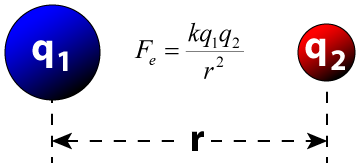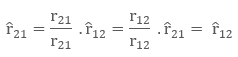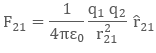> > Coulomb’s Law

# Coulomb’s Law

Did you know that the world exists mainly because of the force of attraction and repulsion? It is mainly because of these attractions and repulsions between particles that the environment remains in a well-equipped and well-balanced form. One such practical application of this theory is the Coulomb’s Law.

Do you know what Coulomb’s Law is all about? Well, in order to find out the extent of repulsion or attraction force between two particles, having some charge, Charles – Augustin de Coulomb came up with the Coulomb’s Law. We will learn about it in this chapter.

### Suggested VideosElectrostatic ForceGauss's law and properties of Gaussian SurfaceElectric Field Lines and its properties## Coulomb’s Law

Coulomb’s Law gives an idea about the force between two point charges. By the word point charge, we mean that in physics, the size of linear charged bodies is very small as against the distance between them. Therefore, we consider them as point charges as it becomes easy for us to calculate the force of attraction/ repulsion between them.Charles-Augustin de Coulomb, a French physicist in 1784, measured the force between two point charges and he came up with the theory that the force is inversely proportional to the square of the distance between the charges. He also found that this force is directly proportional to the product of charges (magnitudes only).

We can show it with the following explanation. Let’s say that there are two charges q1 and q2. The distance between the charges is ‘r’, and the force of attraction/repulsion between them is ‘F’. Then

F  ∝ q1q2

Or, F  ∝  1/r2

F  = k q1q2/ r2

where k is proportionality constant and equals to 1/4 π ε0. Here, ε0 is the epsilon naught and it signifies permittivity of a vacuum. The value of k comes 9 × 109 Nm2/ C2 when we take the S.I unit of value of ε0 is 8.854 × 10-12 C2 N-1 m-2.

According to this theory, like charges repel each other and unlike charges attract each other. This means charges of same sign will push each other with repulsive forces while charges with opposite signs will pull each other with attractive force.

## Vector Form of Coulomb’s Law

The physical quantities are of two types namely scalars (with the only magnitude) and vectors (those quantities with magnitude and direction). Force is a vector quantity as it has both magnitude and direction. The Coulomb’s law can be re-written in the form of vectors. Remember we denote the vector “F” as F, vector r as r and so on.

Let there be two charges q1 and q2, with position vectors r1 and r2 respectively. Now, since both the charges are of the same sign, there will be a repulsive force between them. Let the force on the q1 charge due to q2 be F12 and force on q2 charge due to q1 charge be F21. The corresponding vector from q1 to q2 is r21 vector.

r21 = r2 – r1

To denote the direction of a vector from position vector r1 to  r2 , and from r2 to r1 as:Now, the force on charge q2 due to q1, in vector form is:The above equation is the vector form of Coulomb’s Law.## Remarks on Vector Form of Coulomb’s Law

While applying Coulomb’s Law to find out the force between two point charges, we have to be careful of the following remarks. The vector form of the equation is independent of signs of both the charges, as both the forces are opposite in nature.

The repulsive force F12 , that is the force on charge q1 due to q2 and another repulsive force F21 that is the force on charge q2 due to q1 are opposite in signs, due to change in position vector.

F12 = – F21

This is because the position vector in case of force F12 is r12 and position vector in case of force F21 is r21, now

r21 = r2 – r1

r12 = r1 – r2

Since both r21 and r12 are opposite in signs, they make forces of opposite signs too. This proves that Coulomb’s Law fits into Newton’s Third Law i.e. every action has its equal and opposite reaction. Coulomb’s Law provides the force between two charges when they’re present in a vacuum. This is because charges are free in a vacuum and don’t get interference from other matter or particles.

## Limitations of Coulomb’s Law

Coulomb’s Law is derived under certain assumptions and can’t be used freely like other general formulas. The law is limited to following points:

• We can use the formula if the charges are static ( in rest position)
• The formula is easy to use while dealing with charges of regular and smooth shape, and it becomes too complex to deal with charges having irregular shapes
• The formula is only valid when the solvent molecules between the particle are sufficiently larger than both the charges

## Solved Example for You

Question: Two charges 1 C and – 3 C are kept at a distance of 3 m. Find the force of attraction between them.

Solution: We have q1 = 1C, q2 = – 3C and r = 3m. Then using Coulomb’s Law and substituting above values we get

F = k q1q2/ r2

Or, F = 9 × 109 × 1 × 3/ 32

F = 3 × 109 Newton

Share with friends

## Customize your course in 30 seconds

##### Which class are you in?
5th
6th
7th
8th
9th
10th
11th
12th
Get ready for all-new Live Classes!
Now learn Live with India's best teachers. Join courses with the best schedule and enjoy fun and interactive classes.Ashhar Firdausi
IIT Roorkee
BiologyDr. Nazma Shaik
VTU
ChemistryGaurav Tiwari
APJAKTU
Physics
Get Started

## Browse

##### Electric Charges and FieldsSubscribe
Notify of

## Question Mark?

Have a doubt at 3 am? Our experts are available 24x7. Connect with a tutor instantly and get your concepts cleared in less than 3 steps.